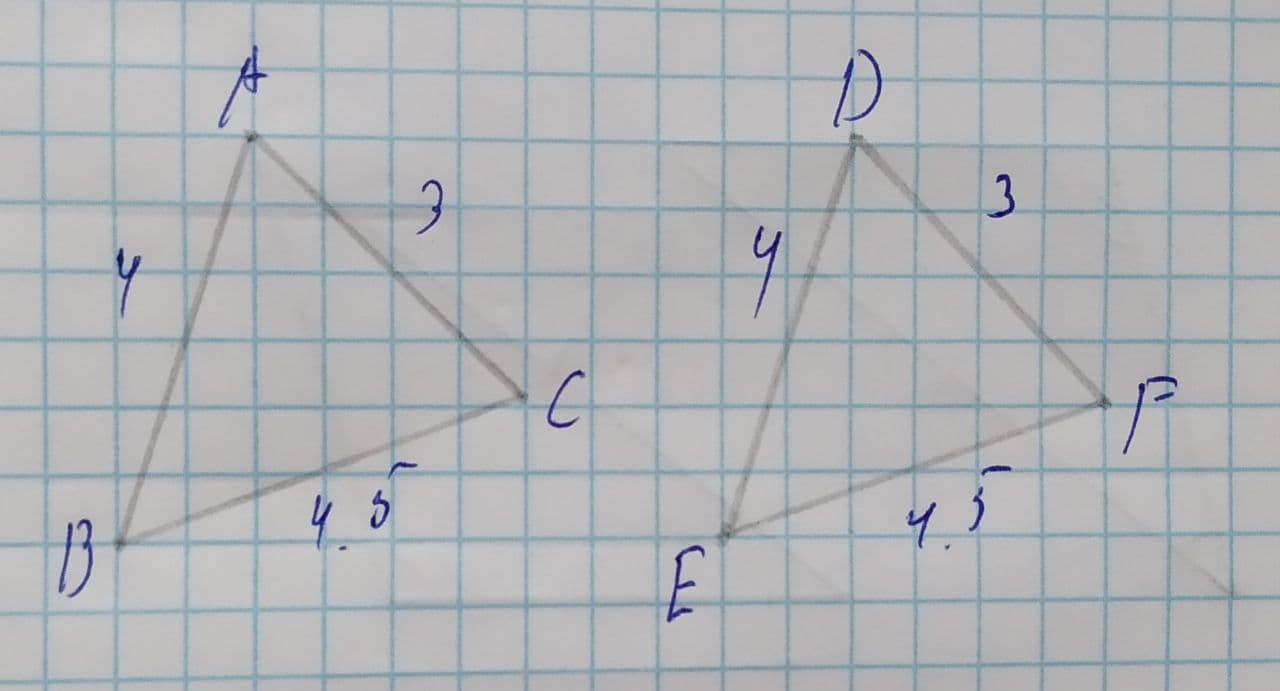#### Didn’t find what you are looking for?

Question# State the congruence relation for \triangle ABC\ and\ \triangle DEF.A.SSSB.SSAC.AAAD.SAS

Polynomial factorization
ANSWEREDState the congruence relation for $$\displaystyle\triangle{A}{B}{C}\ {\quad\text{and}\quad}\ \triangle{D}{E}{F}$$.A.SSS
B.SSA
C.AAA
D.SAS2021-04-19
Step 1
Given,
$$\displaystyle\triangle{A}{B}{C}\ {\quad\text{and}\quad}\ \triangle{D}{E}{F}$$
We have to write the congruence relation for $$\displaystyle\triangle{A}{B}{C}\ {\quad\text{and}\quad}\ \triangle{D}{E}{F}$$
Step 2
In $$\displaystyle\triangle{A}{B}{C}\ {\quad\text{and}\quad}\ \triangle{D}{E}{F}$$
AB=DE=4 (Given)
AC=DF=3 (Given)
BC=EF=4.5 (Given)
Then, From SSS(side-side-side) rule of congruence
$$\displaystyle\triangle{A}{B}{C}\stackrel{\sim}{=}\triangle{D}{E}{F}$$
So,
The correct option is A.